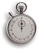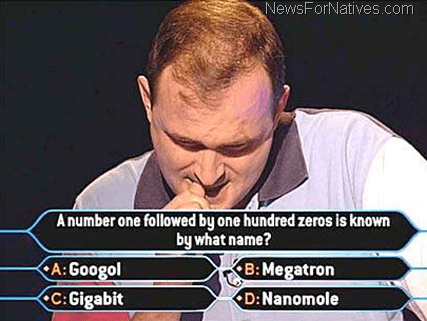How Many Years Is 1 Trillion Seconds

and HOW MANY DAYS ARE IN A MILLION SECONDS and HOW MANY YEARS = 1 BILLION SECONDS

So, just how many years are in a trillion seconds?

One American trillion (ie. 1012) would equal about 31,688 years 269 days 17 hours 34 minutes 25 seconds. (Leap years counted)Specific Answer: 1 Trillion seconds equals 31688.087814028950237026896848937 years!

Numeric Answer: 1,000,000,000,000 Seconds = 31688.087814028950237026896848937 years!

Easy Answer: 1 Trillion seconds is about 32,000 years!

Here is a handy chart for you to know how many zeros are in the biggest numbers!

How many zeros are in various large numbers

 zeros U.S. & scientific community Names of number in other NON USA  countries 1 How many zeros are in ten ten 2 How many zeros are in a hundred hundred 3 How many zeros are in a thousand thousand 6 How many zeros are in a million million 9 How many zeros are in a billion 1000 million (1 milliard) 12 How many zeros are in a trillion billion 15 How many zeros are in a quadrillion 1000 billion 18 How many zeros are in a quintillion trillion 21 How many zeros are in a sextillion 1000 trillion 24 How many zeros are in a septillion quadrillion 27 How many zeros are in a octillion 1000 quadrillion 30 How many zeros are in a nonillion quintillion 33 How many zeros are in a decillion 1000 quintillion 36 How many zeros are in a undecillion sextillion 39 How many zeros are in a duodecillion 1000 sextillion 42 How many zeros are in a tredecillion septillion 45 How many zeros are in a quattuordecillion 1000 septillion 48 How many zeros are in a quindecillion octillion 51 How many zeros are in a sexdecillion 1000 octillion 54 How many zeros are in a septendecillion nonillion 57 How many zeros are in a octodecillion 1000 nonillion 60 How many zeros are in a novemdecillion decillion 63 How many zeros are in a vigintillion 1000 decillion 66 – 120 How many zeros are in a undecillion vigintillion 303 How many zeros are in a centillion centillion 600 10600 41,000 *any How many zeros are in a zillion zillion ????? googol      10^100 googol ????? googolplex 10^googol (10^10^10^2) googolplex ????? n-plex                  10^n n-plex ????? n-minex               10^-n n-minex ????? gesundheit (10^10^10^34) Skewes Number

from http://www.newsfornatives.comThe system used in the U.S. is not as logical as that used in other countries (like Great Britain, France, and Germany). In these other countries, a billion (bi meaning two) has twice as many zeros as a million, and a trillion (tri meaning three) has three times as many zeros as a million, etc.

The scientific community uses the American system of calculating numbers.

FYI: TEN multiplied by itself 600 times gives the figure 1 followed by 600 zeros! This number is so large that it is almost completely beyond our ability to comprehend it!

There are other big numbers with names. A zillion has come to mean an arbitrary or unknown large number. A googol is 10^100. A googolplex is 10^googol (10^10^10^2). This number is too large to write here without exponents. Skewes’ number (gesundheit) is 10^10^10^34 was used as an upper bound in a mathematical proof. Recently 10^10^10^10^10^7 was used in a proof.

The googolplex has given rise to the n-plex notation: n-plex is 10^n. n-minex is 10^-n. Donald Knuth invented arrow notation, where m^n (^ is an up arrow) is the regular m^n. m^^n is m^m^m^m…^m, with n up arrows. m^^^n is m^^m^^m…^^m, with n ^^s. According to The Book of Numbers by J.H.Conway and R.K.Guy, chained arrow notation is the following enhancement: a^^^^^b is written as a>b>5, where > is a right arrow.Google is a play on the word googol, which was coined by Milton Sirotta, nephew of American mathematician Edward Kasner, and was popularized in the book, “Mathematics and the Imagination” by Kasner and James Newman. It refers to the number represented by the numeral 1 followed by 100 zeros. Google’s use of the term reflects the company’s mission to organize the immense, seemingly infinite amount of information available on the web.

How do you write 1 googol?

ONE GOOGOL IS “10000000000000000000000000000000000000000000000000000000000000000000000000000000000000000000000000000”

or “10,000,000,000,000,000,000,000,000,000,000,000,000,000,000,000,000,000,000,000,000,000,000,000,000,000,000,000,000,000,000,000,000,000”